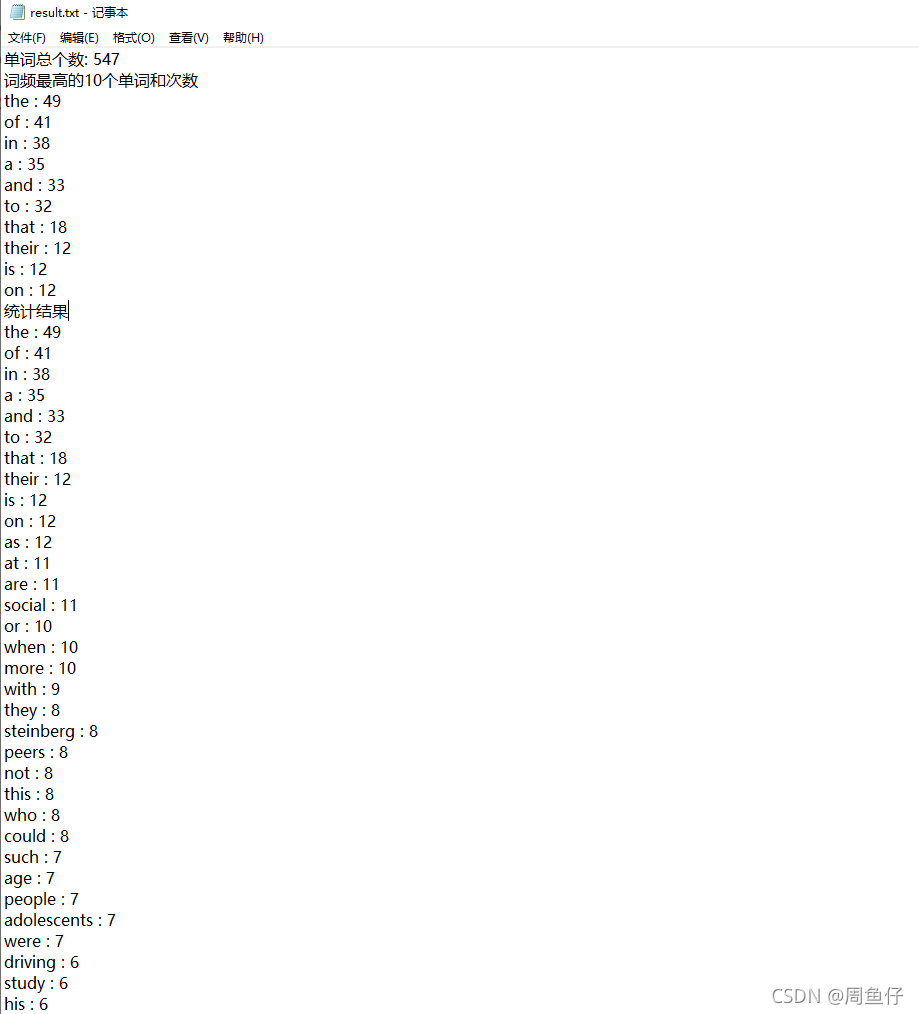# 如何利用python實現詞頻統計功能

## 功能要求

1）從文本中讀入數據：（文件的輸入輸出）

2）不區分大小寫,去除特殊字符。

4）對單詞排序。出現次數

5）輸出詞頻最高的10個單詞和次數

6）把統計結果存入文本

## 方法如下

1.文件的讀取，區分大小寫，去除特殊字符

```import re

def getword():
# 讀取文件
# 將大寫轉化成小寫
# 關閉文件
f.close()
#利用正則除去特殊字符   |\符+
list=re.split('\s+|\,+|\.+|\!+|\:+|\?+|\;+|\(+|\)+|\-+|\_+|\=+|\++|\“+|\、+|\/+|\{+|\}+|\”+|\：+|\。+|\“+|\[+|\]+|\【+|\】+|\—+|\%+|\"+',word)
# 遍歷列表 去除列表中的空格
i = 0
while i < len(list):
if list[i] == '':
list.remove(list[i])
i -= 1
i += 1

# for a in list:
#      if a == "":
#         list.remove(a)
#用for循環的話如果存在多個空字符串 其列表會隨時發生變化，導致無法正常刪除空字符串 所以在使用for…in循環遍歷列表時，最好不要對元素進行增刪操作

#  對於others'優化 如果最後一個字符是‘就將'其去掉
for i in range(len(list)):
l=list[i]
if list[i][-1] == "'":
list[i] = list[i][:-1]
return list

```

2. 統計，排序

```from getfilewords import getword

def statistics():
dict={}  #定義一個空的字典，在後面的運算中逐步添加數據
words=getword()
for word in words: #遍歷整個列表
if word in dict.keys():  #判斷當前單詞是否已經存在 dict.keys()是已存進字典中的單詞
# 補充：keys() 方法用於返回字典中的所有鍵；
# values() 方法用於返回字典中所有鍵對應的值；
#詳情見Test1
dict[word]=dict[word]+1  #在當前單詞的個數上加 1
else:
dict[word]=1  #當前單詞第一次出現時 會把單詞寫入dict字典裡 格式為 ‘單詞'=1
#排序
w_order=sorted(dict.items(),key=lambda x:x,reverse=True)
# print(dict.items())
# dict.items()返回的是列表
# 按字典集合中，每一個元組的第二個元素排列。
# sorted會對dict.items()這個list進行遍歷，把list中的每一個元素，也就是每一個tuple()當做x傳入匿名函數lambda x：x,函數返回值為x
# reverse屬性True為降序 False為升序
return w_order #返回排序後的列表```

3.結果寫入文本

```from WordStatistics import statistics
def writefile():
w_order=statistics()
f = open('result.txt', 'w',encoding='utf-8')
print("文章單詞總個數:",+len(getword()),file=f)
print("文章單詞總個數:", +len(getword()))

# 寫入文件
print("詞頻最高的10個單詞和次數",file=f)
print("詞頻最高的10個單詞和次數")

w_order10=w_order[:10]#將列表的前十位提取並且遍歷 輸出key(單詞)和values(次數)
for key,values in w_order10:
print(key,':',values,file=f)
print(key, ':', values)

#遍歷列表中的所有數據
print("統計結果",file=f)
for key,values in w_order:
print(key,':',values,file=f)
f.close()#關閉文件```

4.程序入口

```import os

from writefile import writefile

print("詞頻統計軟件")
print("正在統計中。。。")
print("統計成功，結果保存到result.txt")
writefile()
print("程序運行結束")
os.system("pause")```

5.運行截圖 這是需要統計的文本## 運行結果